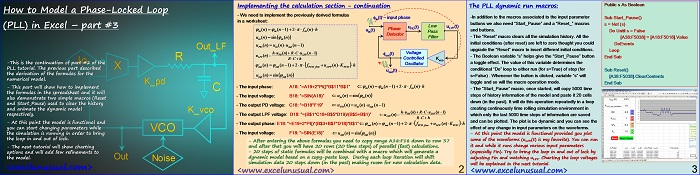# How to Model a Phase-Locked Loop (PLL) in Excel – part#3

This is a continuation of the PLL series of tutorials and it takes the recursive numerical formulas derived in the previous section, implementing a dynamic spreadsheet  model with help from a copy-paste loop type of macro.

This macro emulates the behavior of the phase locked loop model in time. At this point, the model is functional. Charting options for the waveforms will be discussed in the following section.[sociallocker][/sociallocker]

## How to Model a Phase-Locked Loop (PLL) in Excel – part #3

by George Lungu

-This is the continuation of part #2 of the
PLL tutorial. The previous part described X
the derivation of the formulas for the
numerical model.
K_pd

– This part will show how to implement
C
the formulas in the spreadsheet and it will
also demonstrate two simple macros (Reset
and Start_Pause) used to clear the history
and animate the dynamic model
K_vco
respectively.

– At this point the model is functional and
you can start changing parameters while
VCO
the simulation is running in order to bring
the loop in and out of lock.

– The next tutorial will show charting
options and will add few refinements to
the model. O t
Noise

<excelunusual.com>m>

### Implementing the calculation section – continuation

– We need to implement the previously derived formulas
fin(t) – input phase
in a worksheet:

uPD(t) Low uLPF(t)
(n) (n1)2 f (n) h Phase
in in in uin(t)
Pass
Detector
u (n)sin (n) Filter
in in
u (n)  u (n)u (n1)
PD in out
hu (n)RCu (n1) Voltage
PD LPF
u (n)  fout(t)
LPF
RC h Controlled Kvco
Oscillator
(n) (n1)2f u (n)K h
out out VCO_free LPF VCO
uout(t)
u (n)sin  (n)
out

– The input phase: A18: “=A19+2*PI()*B\$11*B\$1”  (n) (n1)2  f (n) h
in in in

– The input voltage: B18: “=SIN(A18)” u (nsin (n)
in in

– The output PD voltage: C18: “=B18*F19” u (n) u (n)u (n1)
PD in out
hu (n)RCu (n1)
PD LPF
– The output LPF voltage: D18: “=(B\$1*C18+B\$5*D19)/(B\$5+B\$1)” u (n)
LPF
RC h

– The output phase: E18: “=E19+2*PI()*(B\$3+B\$7*D18)*B\$1” (n) (n1)2f u (n)K h
out out VCO_free LPF VCO

– The input voltage: F18: “=SIN(E18)” u (n)sin  (n)
out

– After entering the above formulas you need to copy range A18:F18 down to row
37 and after that you will have 20 rows (20 time steps) of parallel (fast) calculations.

– 20 steps of static formulas will be combined with a macro which will generate a
dynamic model based on a copy-paste loop. Each loop iteration will shift the
simulation data 20 steps down (in the past) making room for new calculation data.

### The PLL dynamic run macros:

-In addition to the macros associated to the input parameter buttons we also need “Start_Pause” and a “Reset_” macros and buttons.-The “Reset” macro clears all the simulation history. All the initial conditions (after reset) are left to zero thought you could upgrade the “Reset” macro to insert different initial conditions.

-The Boolean variable “s” helps give the “Start_Pause” button a toggle effect. The value of this variable determines the conditional “Do” loop to either run (for s=True) of stop (for s=False) . Whenever the button is clicked, variable “s” will toggle and so will the macro operation mode.

-The “Start_Pause” macro, once started, will copy 5000 time steps of history information of the model and paste it 20 cells down (in the past).

It will do this operation repeatedly in a loop creating continuously time rolling simulation environment in which only the last 5000 time steps of information are saved and can be plotted.

The plot is be dynamic and you can see the effect of any change in input parameters on the waveforms.

-At this point the model is functional provided you plot some of the waveforms (uLPF(t) is my favorite).

You can run it and while it runs change various input parameters (especially Fin). Try to bring the loop in and out of lock by adjusting Fin and watching uLPF.

Charting the loop voltages will be explained in the next tutorial.

Public s As Boolean

Sub

Start_Pause()s = Not (s)

Do Until s = False[A38:F5038] = [A18:F5018].Value

DoEvents

Loop

End Sub

Sub Reset()

[A38:F5038].ClearContents

End Sub

by George Lungu <www.excelunusual.com>